## 外观和动力受欢迎 数说凯迪拉克ATS-L

2014年11月03日 16:59 来源：www.chextx.com 超过：次关注

在凯迪拉克ATS进口国内9个月之后，上海通用国产凯迪拉克ATS-L正式上市。新车与进口版最大的变化是轴距增加了85mm，相比进口版，国产ATS-L拥有更加充裕的后排空间。这个明显的变化是否为它吸引了更多潜在消费者，而消费者又是如何看待这款新车的呢？请跟随我们一起来看看汽车之家研究中心对凯迪拉克ATS-L的数据调研。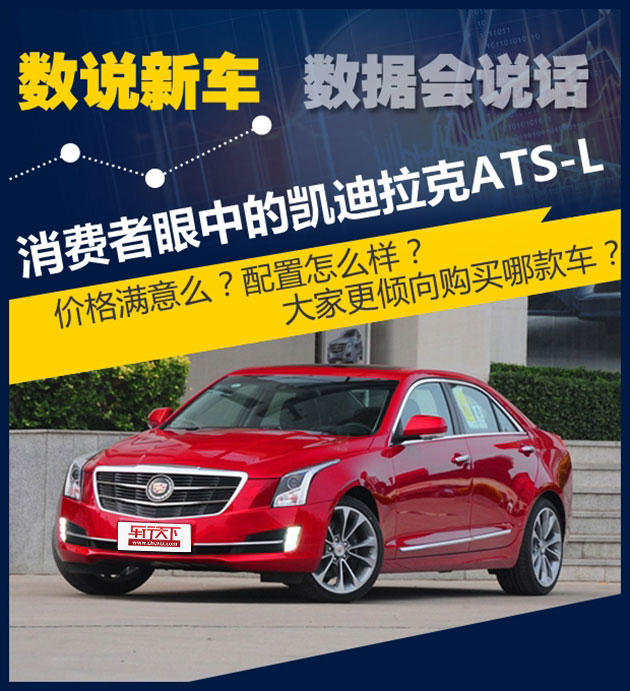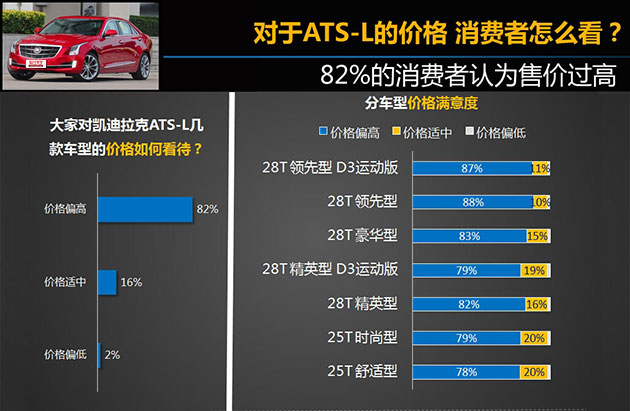汽车之家研究中心在新车上市文章中以问卷投放形式，面向汽车之家用户进行调研，通过回收有效样本，做数据分析。本次报告样本取自2014年8月15日20:00至8月18日13:00期间投放并回收的《通用凯迪拉克ATS-L上市用户调研》，有效样本数为456。

如果您对凯迪拉克ATS-L还不了解，您可以点击以下图片阅读凯迪拉克ATS-L上市文章和试驾文章。

虽然凯迪拉克ATS-L的售价为28.88-46.38万，相比进口版的ATS在起售价有所降低（ATS售价：30.80-43.80万），但仍有82%的网友认为ATS-L的价格偏高。究其原因我们认为，大多数消费者希望国产后的车型售价能够大幅低于进口车型，但是国产ATS-L 28T车型的起售价甚至相比进口版ATS 28T车型价格有所上升，使得一部分消费者对它有些失望。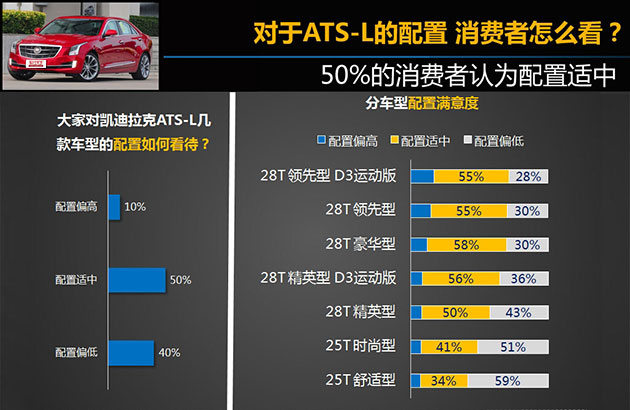从我们统计结果上看，有50%的受访者认为凯迪拉克ATS-L的配置适中，仅有百分之40%的受访者认为配置偏低。从车型配置满意度统计中我们看出，大部分受访者认为ATS-L的25T车型的配置偏低。的确ATS-L 25T车型缺少了消费者喜爱的氙气大灯、中控彩屏等消费者喜爱的配置，对于一款豪华中型车来说缺少这些配置不容易让消费者接受。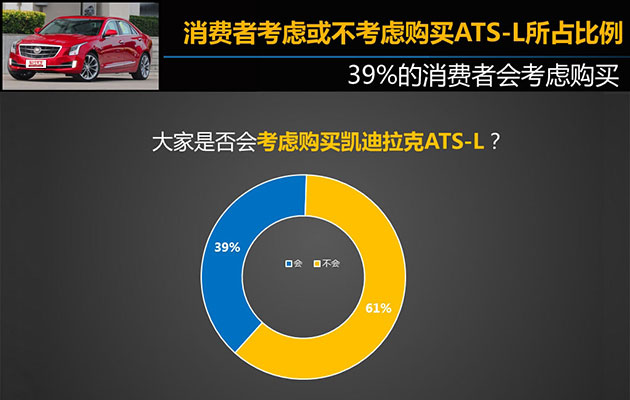接下来我们看一看究竟有多少受访者考虑购买凯迪拉克ATS-L。通过汽车之家研究中心统计，有39%的受访者考虑购买ATS-L，而61%的受访者并不考虑购买ATS-L。考虑到凯迪拉克品牌因素的影响和同级别产品竞争力强等因素，考虑购买新车的人数不是很多也在意料之中。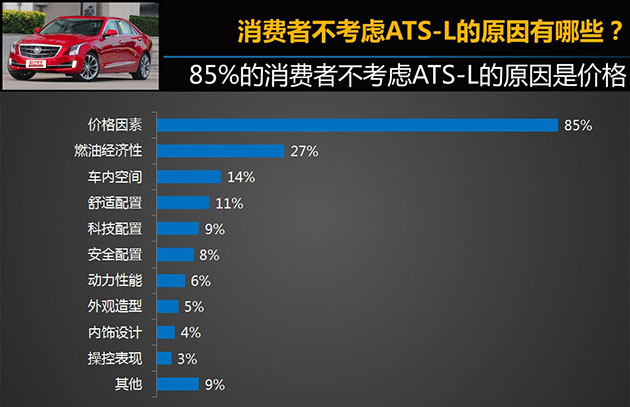从我们的调查数据中看出，85%的受访者认为凯迪拉克ATS-L的价格是他们放弃购买的最大因素，对于一款国产车，它部分车型的售价过高让很多网友表示很难接受。此外，燃油经济性也是受访者放弃选择的因素之一，消费者心中传统美系车型高油耗的固有印象，在短时间内很难改变。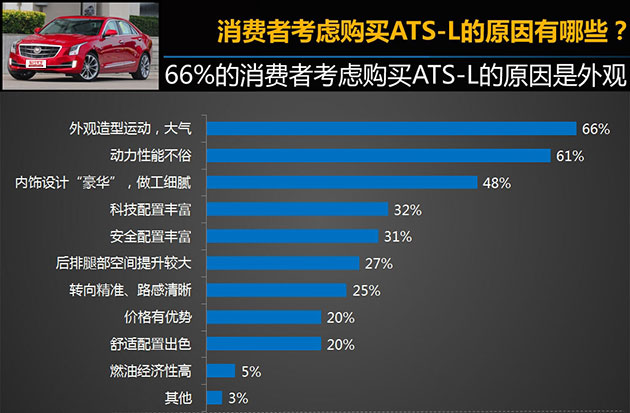通过我们的调查，考虑购买ATS-L的受访者分别有66%和61%喜欢新车运动、大气的外观造型和不俗的动力。的确，凯迪拉克ATS-L的外观造型使用钻石切割设计理念，视觉效果非常运动。此外，全新的2.0T发动机提供两种动力调校，其中28T车型的最大功率更是达到了279马力，扭矩达到400牛·米（相比进口版提升47牛·米），在同级别车型当中这个数据可谓非常傲人。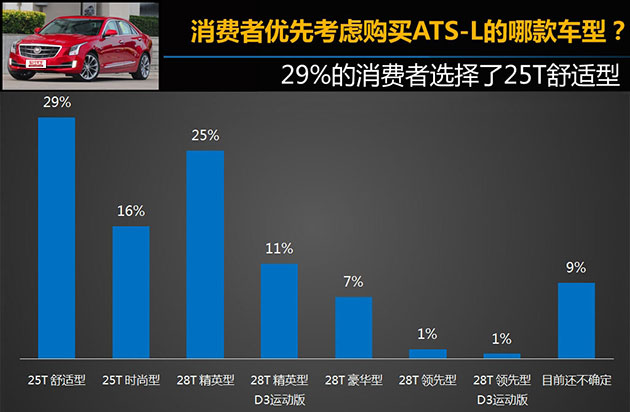通过调查有29%的受访者会优先考虑最低配的25T舒适型，虽然这款车型在配置方面并不太如人意，不过28.88万的售价确实有着不小的诱惑力。此外，还有25%的受访者会优先购买28T精英型，这款车是搭载2.0T高功率发动机的最低配车型，虽然配置并不高，但279马力的动力输出让不少喜爱运动的消费者为之心动。结合我们编辑撰写的《凯迪拉克ATS-L购车手册》我们发现，ATS-L 25T舒适型和28T精英型名列推荐车型的第二名和第一名，与消费者优先考虑车型基本保持一致。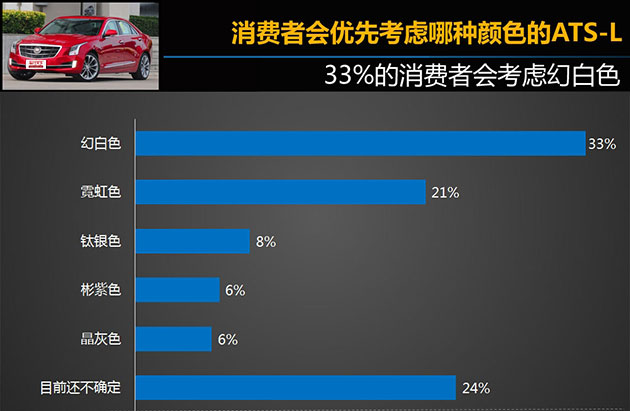颜色选择方面，考虑购买凯迪拉克ATS-L的受访者选择白色车漆的人数最多，这些消费者可能是考虑到在日常的使用当中，白色车身比较耐脏，又能够给人一种大气的视觉效果。除此之外，凯迪拉克ATS-L主打的红色也是消费者主要考虑的颜色之一，不过还有24%的受访人群表示并不确定究竟会选择哪种颜色。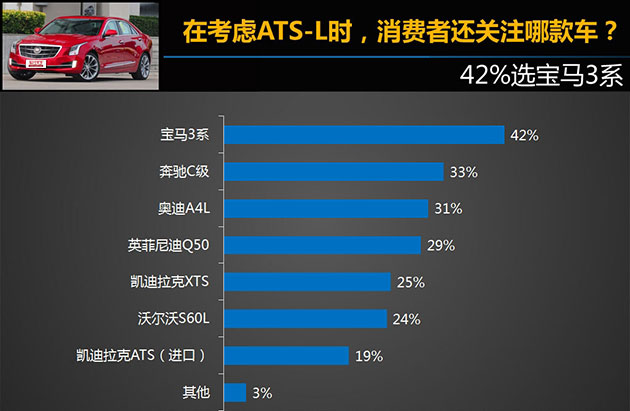通过调查我们发现，受访者在考虑凯迪拉克ATS-L的同时还在考虑宝马3系。的确，从凯迪拉克ATS诞生之时，厂家就把宝马3系视为新车的最大竞争对手，两款车型都是主打运动性的豪华中型车。此外奔驰C级、奥迪A4L以及英菲尼迪Q50也是较多消费者可能会考虑的车型。

通过我们的调查发现，凯迪拉克ATS-L还是受到了不少消费者的关注，作为凯迪拉克主打运动的车型之一，ATS-L凭借运动的造型和澎湃的动力获得了不少消费者的芳心。此外，轴距的加长也为新车内部空间增色不少。未来新车的最大竞争者将是同样主打运动的宝马3系Li，不过随着奔驰国产C级长轴距版和Q50L即将上市，凯迪拉克ATS-L的竞争对手将越来越多，它究竟能否在豪华中型车市场站稳脚跟，我们还要等待市场的检验。

#### 相关文章

﻿
• 快速找车
• 选择品牌
• 选择品牌
• A  奥迪
• A  阿斯顿·马丁
• A  阿尔法·罗密欧
• B  宝沃
• B  布加迪
• B  巴博斯
• B  保时捷
• B  宾利
• B  奔驰
• B  宝马
• B  本田
• B  别克
• B  标致
• B  比亚迪
• B  宝骏
• B  北汽制造
• B  北汽新能源
• B  北汽幻速
• B  北汽威旺
• B  北京汽车
• B  奔腾
• B  北汽绅宝
• B  北汽昌河
• C  长安欧尚
• C  长安
• C  长安凯程
• C  长城
• D  大众
• D  道奇
• D  DS
• D  东南
• D  东风风神
• D  东风风行
• D  东风小康
• D  东风风度
• D  东风
• F  福特
• F  丰田
• F  菲亚特
• F  法拉利
• F  福田
• F  福迪
• F  福汽启腾
• G  观致
• G  广汽传祺
• G  广汽吉奥
• G  GMC
• H  红旗
• H  汉腾汽车
• H  哈弗
• H  哈飞
• H  海格
• H  海马
• H  华颂
• H  黄海
• H  华泰
• H  恒天
• J  几何汽车
• J  捷达
• J  吉利汽车
• J  捷豹
• J  Jeep
• J  江淮
• J  江铃
• J  金杯
• J  九龙
• J  金旅
• K  凯翼
• K  凯迪拉克
• K  克莱斯勒
• K  科尼塞克
• K  卡威
• K  开瑞
• L  路虎
• L  林肯
• L  劳斯莱斯
• L  兰博基尼
• L  雷克萨斯
• L  铃木
• L  领克
• L  雷诺
• L  理念
• L  力帆
• L  莲花汽车
• L  猎豹
• L  路特斯
• L  陆风
• M  马自达
• M  MG
• M  MINI
• M  玛莎拉蒂
• M  摩根
• M  迈凯轮
• N  纳智捷
• O  欧拉
• O  欧宝
• O  讴歌
• O  欧朗
• Q  奇瑞
• Q  起亚
• Q  启辰
• R  日产
• R  荣威
• R  瑞麒
• S  SERES赛力斯
• S  三菱
• S  斯威汽车
• S  萨博
• S  smart
• S  斯柯达
• S  斯巴鲁
• S  思铭
• S  双龙
• S  上汽大通
• S  双环
• T  特斯拉
• T  腾势
• W  蔚来
• W  沃尔沃
• W  WEY
• W  五菱汽车
• W  五十铃
• W  威兹曼
• W  威麟
• X  现代
• X  雪佛兰
• X  星途
• X  雪铁龙
• X  小鹏汽车
• X  西雅特
• Y  一汽
• Y  英菲尼迪
• Y  英致
• Y  依维柯
• Y  野马汽车
• Y  永源
• Z  众泰
• Z  中华
• Z  中兴
• Z  知豆
• 选择车系
• 选择车系
• 车型对比
• 选择品牌
• 选择品牌
• A  奥迪
• A  阿斯顿·马丁
• A  阿尔法·罗密欧
• B  宝沃
• B  布加迪
• B  巴博斯
• B  保时捷
• B  宾利
• B  奔驰
• B  宝马
• B  本田
• B  别克
• B  标致
• B  比亚迪
• B  宝骏
• B  北汽制造
• B  北汽新能源
• B  北汽幻速
• B  北汽威旺
• B  北京汽车
• B  奔腾
• B  北汽绅宝
• B  北汽昌河
• C  长安欧尚
• C  长安
• C  长安凯程
• C  长城
• D  大众
• D  道奇
• D  DS
• D  东南
• D  东风风神
• D  东风风行
• D  东风小康
• D  东风风度
• D  东风
• F  福特
• F  丰田
• F  菲亚特
• F  法拉利
• F  福田
• F  福迪
• F  福汽启腾
• G  观致
• G  广汽传祺
• G  广汽吉奥
• G  GMC
• H  红旗
• H  汉腾汽车
• H  哈弗
• H  哈飞
• H  海格
• H  海马
• H  华颂
• H  黄海
• H  华泰
• H  恒天
• J  几何汽车
• J  捷达
• J  吉利汽车
• J  捷豹
• J  Jeep
• J  江淮
• J  江铃
• J  金杯
• J  九龙
• J  金旅
• K  凯翼
• K  凯迪拉克
• K  克莱斯勒
• K  科尼塞克
• K  卡威
• K  开瑞
• L  路虎
• L  林肯
• L  劳斯莱斯
• L  兰博基尼
• L  雷克萨斯
• L  铃木
• L  领克
• L  雷诺
• L  理念
• L  力帆
• L  莲花汽车
• L  猎豹
• L  路特斯
• L  陆风
• M  马自达
• M  MG
• M  MINI
• M  玛莎拉蒂
• M  摩根
• M  迈凯轮
• N  纳智捷
• O  欧拉
• O  欧宝
• O  讴歌
• O  欧朗
• Q  奇瑞
• Q  起亚
• Q  启辰
• R  日产
• R  荣威
• R  瑞麒
• S  SERES赛力斯
• S  三菱
• S  斯威汽车
• S  萨博
• S  smart
• S  斯柯达
• S  斯巴鲁
• S  思铭
• S  双龙
• S  上汽大通
• S  双环
• T  特斯拉
• T  腾势
• W  蔚来
• W  沃尔沃
• W  WEY
• W  五菱汽车
• W  五十铃
• W  威兹曼
• W  威麟
• X  现代
• X  雪佛兰
• X  星途
• X  雪铁龙
• X  小鹏汽车
• X  西雅特
• Y  一汽
• Y  英菲尼迪
• Y  英致
• Y  依维柯
• Y  野马汽车
• Y  永源
• Z  众泰
• Z  中华
• Z  中兴
• Z  知豆
• 选择车系
• 选择车系
• 选择车型
• 选择车型
• 意见反馈